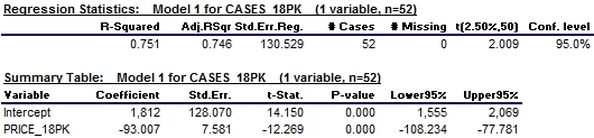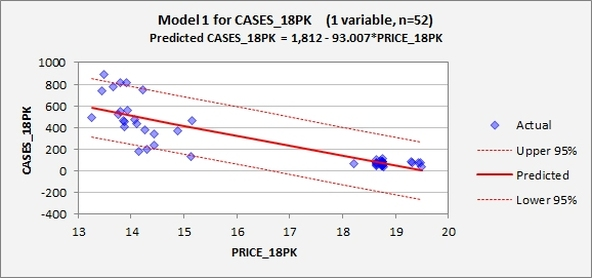When you have a set of data points you can adjust a line to them with a regression.

In a linear regression a line is adjusted to the points and the slope and interception of the line is calculated.

If you use Excel you may like to use also this free addon

http://regressit.comIt creates graphs of the linear regression with a 95% confidence interval.## Analysis of linear regression

The quality of the adjustment can be seen from the plot or from values of R^2.